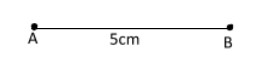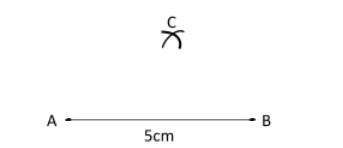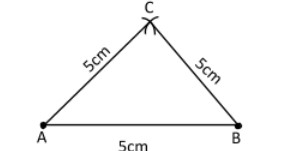QuestionAnswers

# Explain how to construct an equilateral $\Delta ABC$ of side 5cm, with the help of compass and ruler solutionVerified
130.5k+ views
Hint: Equilateral triangle means all the sides in a triangle are equal in length and each angle is ${60^o}$.

Complete step by step solution:
Let us suppose $\Delta ABC$is an equilateral triangle in which $AB = BC = CA = 5cm$
(i) Draw a line segment $AB = 5cm$(ii) With centres $A$and $B$and radius $5cm$ each. Draw two arcs one from point $A$and other from point $B$.(iii) Joint $AC$and $BC$Therefore, the required $\Delta ABC$ is an equilateral triangle with each side$5cm$.

Note:When constructing triangles; make sure to do it in accordance to the given condition and;if it has been asked to construct using ruler and compass do it using the same method and do not use protractor for the same